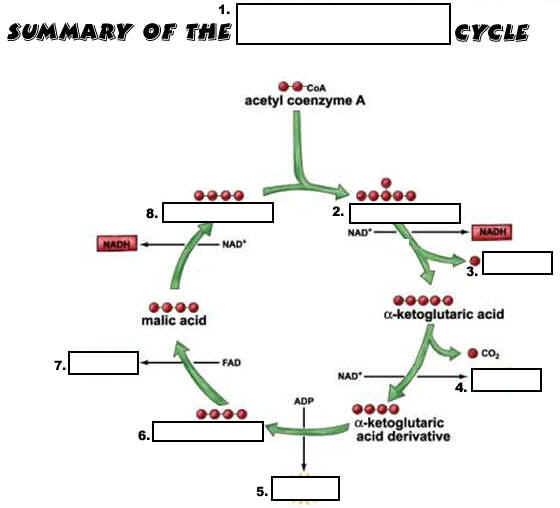# Cell Respiration PPT Q-B1

ADP, ATP, & Cellular Respiration
PowerPoint Question Guide

1. _______ is the energy used by all cells.

2. ATP stands for ________________ ______________.

3. ATP is an ___________ molecule containing high-energy ____________ bonds.

4. The sugar in ATP is ____________, while the nitrogen base is ___________.

5. How many phosphate groups does ATP contain?

6. How do we get energy from ATP?

7. Make a simple sketch of ATP and show the high-energy bond that is broken.

8. To break the last phosphate bond in ATP, _________ must be added.

9. The process is called ___________.

10. What enzyme is used to help weaken & break the last phosphate bond in ATP?

11. Can ATP be remade?

12. When the last phosphate bond of ATP is broken, __________ and a free __________ form.

13. What enzyme can be used to rejoin ADP and a free phosphate to make more ATP?

14. Using ATP’s energy and then remaking it is called the ________________ cycle.

15. In the body, ATP is made during the process of _____________ ________________.

16. Cellular respiration takes place in both ____________ and ____________.

17. Cellular respiration requires the gas ____________.

18. In cellular respiration, _____________ is oxidized (loses electrons) and ___________ is reduced (gains electrons).

19. The breakdown of one glucose molecule results in ________ to _______ ATP molecules of energy.

20. Write the overall equation for cellular respiration.

21. Cellular respiration is an example of a ________ reaction.

22. REDOX stands for _____________-____________ reactions.

23. What are the products of cellular respiration?

24. What carries the energized electrons from glucose in cellular respiration?

25. NAD+_ is a _____________ that forms ____________ when it is reduced (picks up electrons).

26. What does NAD+ stand for?

27. Name a second coenzyme that acts as an energy carrier in cellular respiration.

28. What does FAD+ stand for?

29. FAD+ becomes __________ whenever it is reduced.

30. Cellular respiration like photosynthesis is a _______________ ____________ because it involves many reactions to make or break down carbohydrates.

31. Cellular respiration is an ______________ reaction because it releases energy from glucose.

32. Glucose is broken down into __________ and _________.

33. Is cellular respiration catabolic or anabolic? explain why.

34. Name the 3 stages of cellular respiration.

35. ____________ takes place in the cytoplasm of cells., while the __________ cycle and ETC take place in the _______________.

36. Sketch and label the parts of a mitochondrion.

37. Describe the outer surface of the mitochondria.

38. The inner membrane of the mitochondria is ___________.

39. The folds of the inner mitochondrial membrane are called ___________.

40. The innermost space of the mitochondria is known as the ___________.

41. Using the PowerPoint diagram, answer the following:

a. Glycolysis occurs where?

b. Glycolysis produces what energy molecule?

c. The products of glycolysis enter what other part of a cell?

d. What organic compound enters the Krebs cycle?

e. Electron carriers (NADH & FADH) carry electrons to what?

f. The ETC occurs across what?

g. What is the product at the end of the ETC?

h. What gas is added at the end of the ETC?

i. The Krebs cycle occurs where?

j. What gas is a product of the Krebs cycle?

k. Is ATP made in the Krebs cycle?

l. Is ATP in the ETC?

42. Is glycolysis aerobic or anaerobic? Explain why.

43. Glycolysis requires how much ATP to get started?

44. In glycolysis, glucose is split into two molecules of ______________ or pyruvic _________.

45. Is any CO2 produced during glycolysis?

46. Glycolysis uses 2 ATP and produces ______ ATP.

47. How much NADH (energy carrier) is made during glycolysis?

48. Glycolysis does ______ require oxygen but may occur if _________ is present.

49. If oxygen is NOT present, the products of glycolysis enter a process called _______________.

50. Fermentation is an _____________ process because no ___________ is needed.

51. Name the 2 types of fermentation.

52. Which fermentation occurs in tired muscle cells?

53. _________ acid builds up and makes muscles feel tired.

54. ____________ fermentation in yeasts produces an alcohol called _____________.

55. Fermentation only nets ______ molecules of ATP.

56. Why did Hans Krebs receive the Nobel Prize in medicine in 1953?

57. Why did he have to leave Germany before WWII?

58. Does the Krebs cycle need oxygen?

59. Processes needing oxygen are said to be ____________.

60. How many turns of the Krebs cycle are needed to burn one molecule of glucose?

61. What gas is made during the Krebs Cycle?

62. Where does the Krebs cycle take place?

63. TWO TURNS of the Krebs cycle produces ______ CO2 molecules, ______ NADH, _____FADH, and ____ ATP molecules.

64. Label the parts of the Krebs Cycle.65. The ETC occurs across the inner membrane of the ____________ and produces ________ as an end product.

66. The ETC uses the energized electrons carried by the coenzymes __________ and ________ to make 34 ATP’s of energy.

67. Each NADH makes _______ ATP’s, while each FADH makes only ______ ATP’s.